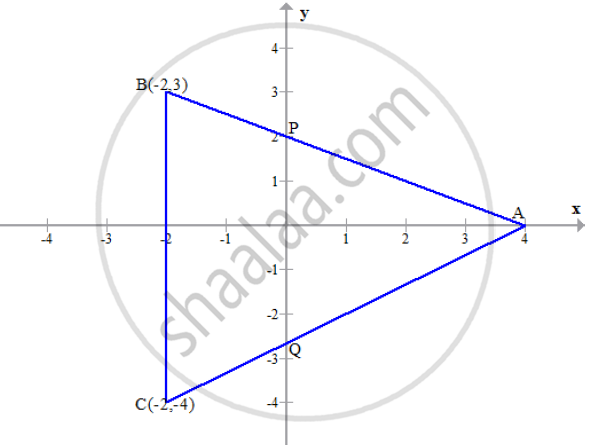Share

# In the Given Figure Abc is a Triangle and Bc is Parallel to the Y-axis. Ab and Ac Intersect the Y-axis at P and Q Respectively. Write the Coordinates of A. Find the Length of Ab and Ac Find the Ratio in Which Q Divides Ac. Find the Equation of the Line Ac - ICSE Class 10 - Mathematics

ConceptGeometric Understanding of c as the y-intercept Or the Ordinate of the Point Where the Line Intercepts the y Axis Or the Point on the Line Where x=0

#### Question

In the given figure ABC is a triangle and BC is parallel to the y-axis. AB and AC intersect
the y-axis at P and Q respectively.1) Write the coordinates of A.

2) Find the length of AB and AC

3) Find the ratio in which Q divides AC.

4) Find the equation of the line AC

#### Solution

1) The line intersects the x-axis where, y = 0. Thus, the coordinates of A are (4, 0).

2) Length of AB = sqrt((4-(-2))^2+ (0 -3)^2) = sqrt(36 + 9) = sqrt45 = 3sqrt5units

3) Let Q divides AC in the ratio m1 : m2. Thus, the co-ordinates of Q are (0, y)

Since x = (m_1x_2 + m_2x_1)/(m_1 + m_2)

=> 0 = (m_1 (-2) + m_2(4))/(m_1 + m_2) => 2m_1 = 4m_2 => m_1 = 2m_2

=> m_1/m_2 = 2/1

∴ Required ratio is  2 : 1

4) A(4, 0) = A(x1,y1) and B(-2,-4) = b(x2, y2)

Slope of AC = (-4-0)/(-2-4) = (-4)/(-6) = 2/3

∴ Equation of line AC is given by y - y_1 = m(x - x_1)

=> y - 0 = 2/3 (x - 4)

=> 3y = 2x - 8

=> 2x - 3y = 8

Is there an error in this question or solution?

#### APPEARS IN

2014-2015 (March) (with solutions)
Question 6.2 | 4.00 marks
Solution In the Given Figure Abc is a Triangle and Bc is Parallel to the Y-axis. Ab and Ac Intersect the Y-axis at P and Q Respectively. Write the Coordinates of A. Find the Length of Ab and Ac Find the Ratio in Which Q Divides Ac. Find the Equation of the Line Ac Concept: Geometric Understanding of c as the y-intercept Or the Ordinate of the Point Where the Line Intercepts the y Axis Or the Point on the Line Where x=0.
S